Published

# Peak Quality

Share the cool but maintain your own cool during peak energy usage!

IntermediateFull instructions provided2 hours728## Things used in this project

### Hardware componentsParticle Photon
×1Resistor 10k ohm
×1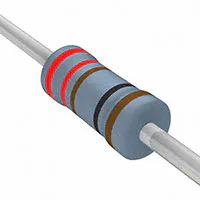Resistor 221 ohm
×1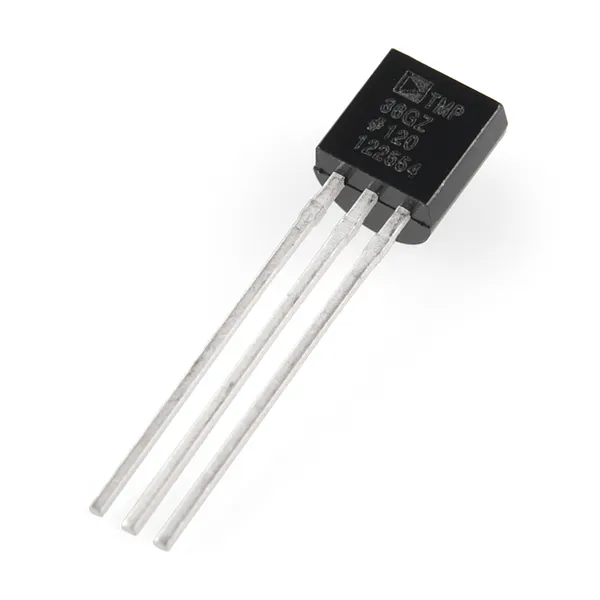Temperature Sensor
×1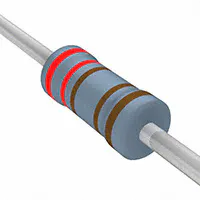Resistor 2.21k ohm
×1Capacitor 100 µF
×1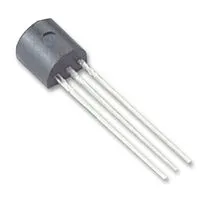General Purpose Transistor NPN
×2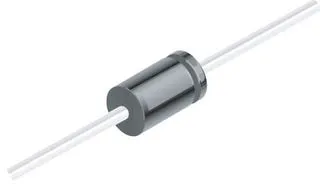1N4007 – High Voltage, High Current Rated Diode
×2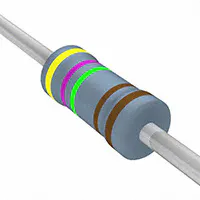Resistor 4.75k ohm
×1
 Relay (generic)
×1×1Jumper wires (generic)
×1

### Software apps and online servicesIFTTT Maker service

## Schematics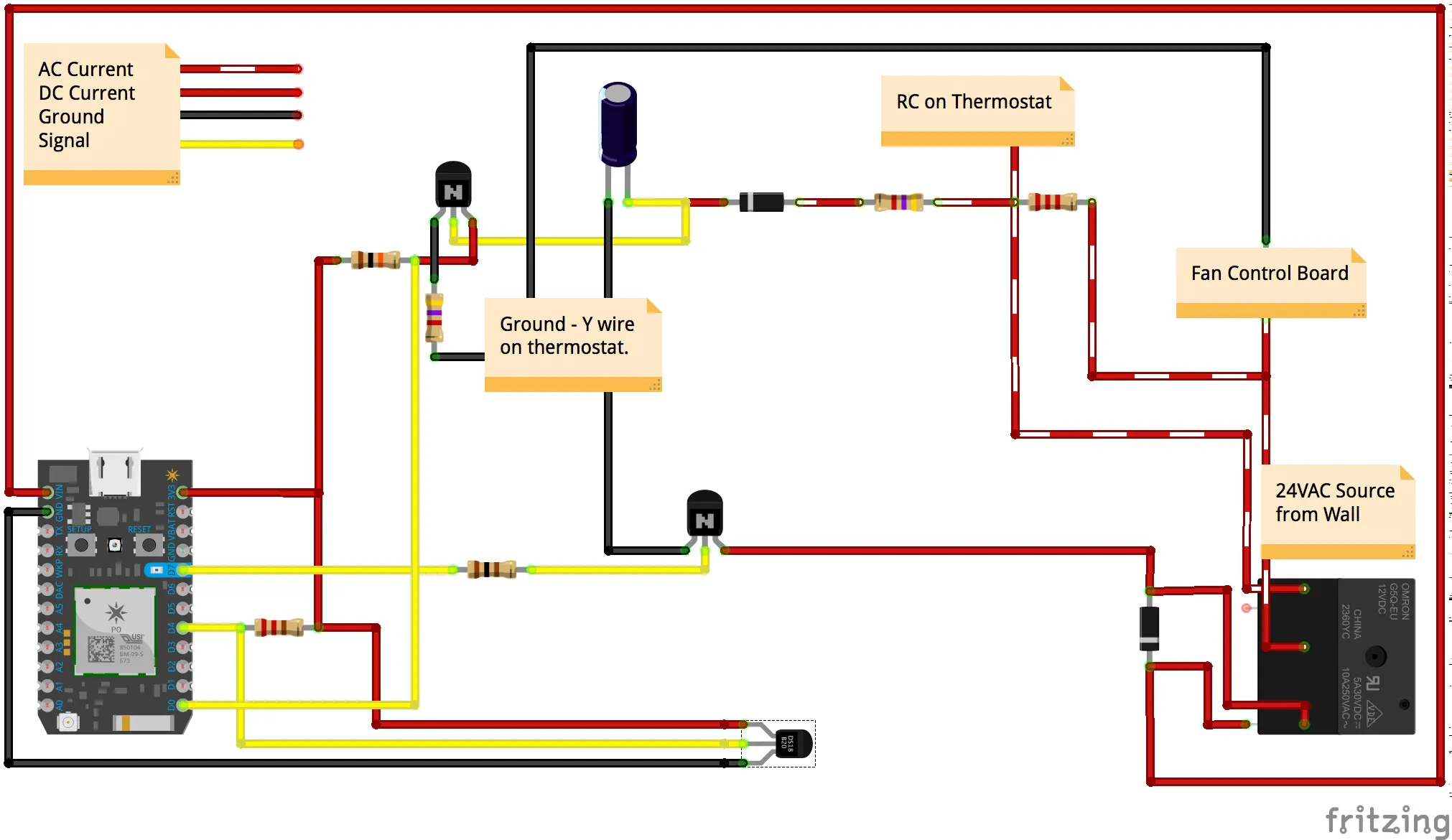### Schematic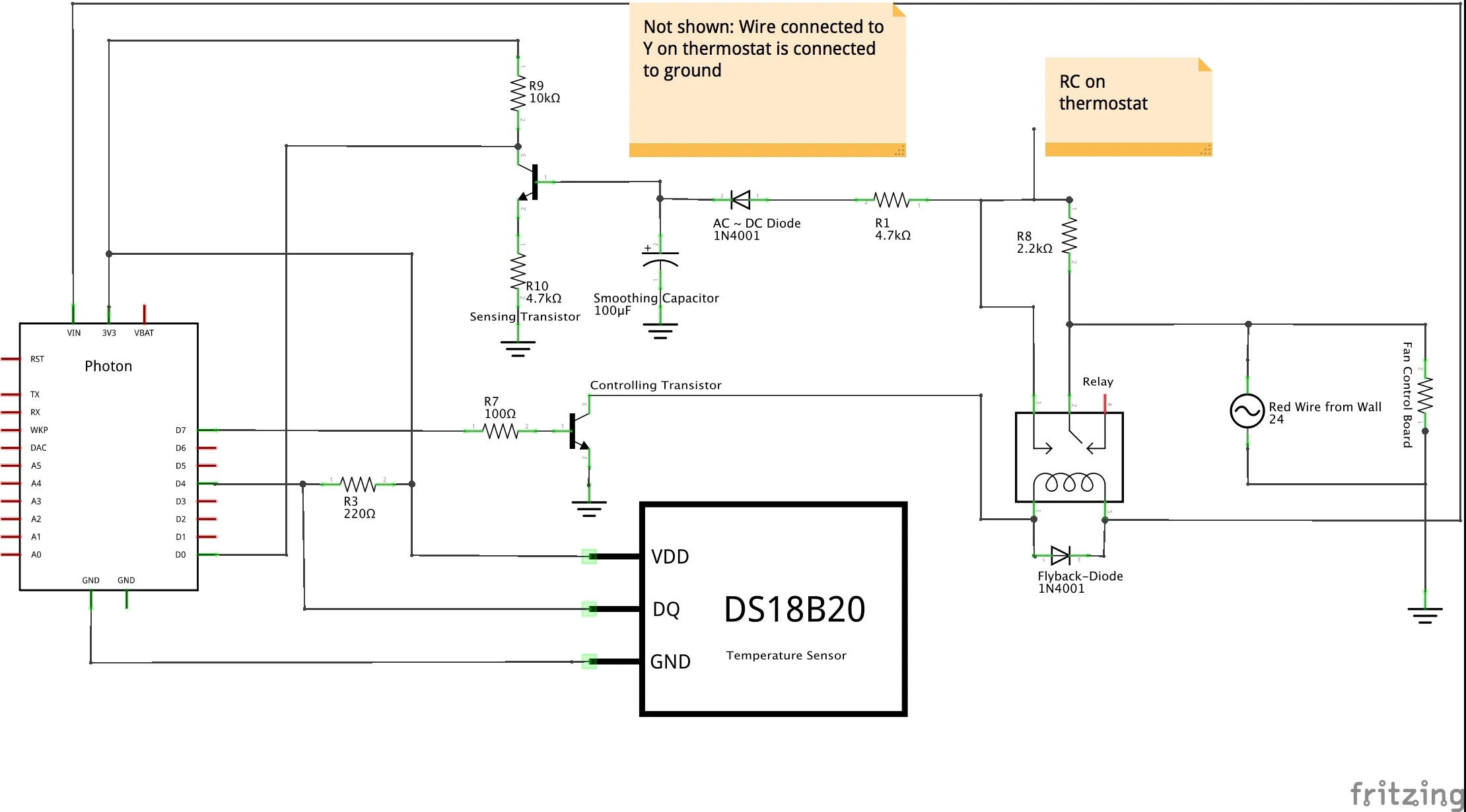## Code

### GATEKEEPER.INO

C/C++
This code includes the conversation outline necessary to decide with thermostat is allowed to run. This code runs on both Photons involved.
```// This #include statement was automatically added by the Particle IDE.
#include "OneWire/OneWire.h"

OneWire ds = OneWire(D4);  // 1-wire signal on pin D4

String localDataString; // strings that will be populated for personal datalogging
String foreignDataString;
String prevLocalData;
String prevForeignData;

int setPoint;
int error = 0;

int myTemp; // This is the local temperature
int myDev; // Calculated deviation from setpoint
bool myCall = false; // Whether or not the thermostat is trying to turn on
bool myRun = false; // whether the ac is allowed to run
int myPriority; // status of priority
int myReq; // used to request priority from foreign member
int myAns; // used to answer requests from foreign memeber

bool prevRun; // used to decide when to activate timer
int prevTemp; // used for error-checking

// variables from foreign member that mirror local data
int forTemp;
int forDev;
bool forCall;
bool forRun;
bool forPriority;
int forReq;
int forAns;
int forTimer;

Timer oneMin(30000, everyMinute);
Timer thirtyMinutes(1800000, thirtyMinutesPassed, true);

SYSTEM_MODE(AUTOMATIC);

void setup() {

Serial.begin(9600);

setPoint = 75;

oneMin.start(); // start the timer that calls the function for posting logged data

pinMode(D7, OUTPUT); // Relay (LOW = block)
pinMode(D0, INPUT_PULLUP); // Thermostat call (LOW = no call)
pinMode(D2, OUTPUT);

digitalWrite(D2, HIGH);

// Each unit will send publish different types of data, but they will be in a single subscribed event
// Since these events are public, a random 8-digit number is assigned to each member in the system for uniqueness for event name
// letter at the end of even name discerns the two photons
Particle.subscribe("32420324_A", foreignDataHandler);

// Monitor variables for debugging
Particle.variable("myRun", myRun);
Particle.variable("Temperature", myTemp);
}

void loop() {

myCall = false;
}
else if (digitalRead(D0) == HIGH) {
myCall = true;
}

if (myPriority == 0) {
digitalWrite(D7, LOW);
myRun = 0;
delay(50);
}

if (myRun == true) {
digitalWrite(D0, HIGH); // activate relay to AC signal
}
else digitalWrite(D0, LOW); // de-activate relay to AC signal

// When something in the local or foreign data string changes, it is published
if (strcmp(prevLocalData,localDataString) != 0) {
Particle.publish("local_GateKeeper", localDataString, PRIVATE);
delay(500);
}

if (strcmp(prevForeignData,foreignDataString) != 0) {
Particle.publish("Foreign_GateKeeper", foreignDataString, PRIVATE);
delay(500);
}

// comparison values for next loop
prevLocalData = localDataString;
prevForeignData = foreignDataString;
}

void everyMinute() {

// Get current temperature and then println
// getCurrentTemp returns nothing, but sets varaible temperature in the function
getCurrentTemp();
delay(50);

while (error == 1) {
getCurrentTemp();
delay(50);
}

// Calculates and prints the current setpoint deviation
// Will be used for priority assignment
myDev = abs(myTemp - setPoint);

/**************************************************Requests******************************************/
// local thermostat calls to run, but isnt running
if (myCall == true && myRun == false) {
if (myPriority == false) {
if (forAns == 0 || forAns == -1) {
myReq = 1; //make request - ask if it's okay to turn on
}
//foreign member approves local priority
else if (forAns == 1) {
myPriority = true;
}
}
else {
myRun = true; // if local already has priority
myReq = 0; // if local has priority, no need for requests
}
}

// if local thermostat is idle
if (myCall == false) {
myReq = 0;
myRun = false;
}

// Check for okay to start running the AC
if (myRun == true && myPriority == true) {
myReq = 0;
if (prevRun == false && myRun == true) {
thirtyMinutes.start(); //thiry minute timer starts
}
}

// Put together the data to be published
// Tilde acts as a delimiter so all data can be published in a single string and broken apart later
localDataString = String(myTemp) + "~" + String(myDev) + "~" + String(myCall) + "~" + String(myRun) + "~" + String(myPriority) + "~" + String(myReq) + "~" + String(myAns) + "~" + String(thirtyMinutes.isActive());

Particle.publish("32420324_E", localDataString);
delay(500);

prevRun = myRun;
}

// Code copied from ONEWIRE library to read temperature from single-wire temperature probe
// Much of the troubleshooting println commands have been commented out
void getCurrentTemp() {

byte i;
byte present = 0;
byte type_s;
byte data;
float celsius, fahrenheit;
float lastTemp;

//Serial.println();
ds.reset_search();
delay(250);
return;
}

// The order is changed a bit in this example
// first the returned address is printed

//Serial.print("ROM =");
for( i = 0; i < 8; i++) {
//Serial.write(' ');
}

// second the CRC is checked, on fail,

Serial.println("CRC is not valid!");
return;
}
Serial.println();

// we have a good address at this point
// what kind of chip do we have?
// we will set a type_s value for known types or just return

// the first ROM byte indicates which chip
case 0x10:
//Serial.println("  Chip = DS1820/DS18S20");
type_s = 1;
break;
case 0x28:
// Serial.println("  Chip = DS18B20");
type_s = 0;
break;
case 0x22:
//Serial.println("  Chip = DS1822");
type_s = 0;
break;
case 0x26:
//Serial.println("  Chip = DS2438");
type_s = 2;
break;
default:
//Serial.println("Unknown device type.");
return;
}

// this device has temp so let's read it

ds.reset();               // first clear the 1-wire bus
ds.select(addr);          // now select the device we just found
// ds.write(0x44, 1);     // tell it to start a conversion, with parasite power on at the end
ds.write(0x44, 0);        // or start conversion in powered mode (bus finishes low)

// just wait a second while the conversion takes place
// different chips have different conversion times, check the specs, 1 sec is worse case + 250ms
// you could also communicate with other devices if you like but you would need

delay(1000);     // maybe 750ms is enough, maybe not, wait 1 sec for conversion

// we might do a ds.depower() (parasite) here, but the reset will take care of it.

// first make sure current values are in the scratch pad

present = ds.reset();
ds.write(0xB8,0);         // Recall Memory 0
ds.write(0x00,0);         // Recall Memory 0

present = ds.reset();
if (type_s == 2) {
ds.write(0x00,0);       // The DS2438 needs a page# to read
}

// transfer and print the values

//Serial.print("  Data = ");
//Serial.print(present, HEX);
//Serial.print(" ");
for ( i = 0; i < 9; i++) {           // we need 9 bytes
//Serial.print(data[i], HEX);
//Serial.print(" ");
}
//Serial.print(" CRC=");
// Serial.print(OneWire::crc8(data, 8), HEX);
//Serial.println();

// Convert the data to actual
// because the result is a 16 bit signed integer, it should
// be stored to an "int16_t" type, which is always 16 bits
// even when compiled on a 32 bit processor.
int16_t raw = (data << 8) | data;
if (type_s == 2) raw = (data << 8) | data;
byte cfg = (data & 0x60);

switch (type_s) {
case 1:
raw = raw << 3; // 9 bit resolution default
if (data == 0x10) {
// "count remain" gives full 12 bit resolution
raw = (raw & 0xFFF0) + 12 - data;
}
celsius = (float)raw * 0.0625;
break;
case 0:
// at lower res, the low bits are undefined, so let's zero them
if (cfg == 0x00) raw = raw & ~7;  // 9 bit resolution, 93.75 ms
if (cfg == 0x20) raw = raw & ~3; // 10 bit res, 187.5 ms
if (cfg == 0x40) raw = raw & ~1; // 11 bit res, 375 ms
// default is 12 bit resolution, 750 ms conversion time
celsius = (float)raw * 0.0625;
break;

case 2:
data = (data >> 3) & 0x1f;
if (data > 127) {
celsius = (float)data - ((float)data * .03125);
}else{
celsius = (float)data + ((float)data * .03125);
}
}

// remove random errors
if((((celsius <= 0 && celsius > -1) && lastTemp > 5)) || celsius > 125) {
celsius = lastTemp;
}

fahrenheit = celsius * 1.8 + 32.0;
lastTemp = celsius;

/*
Serial.print("  Temperature = ");
Serial.print(celsius);
Serial.print(" Celsius, ");
Serial.print(fahrenheit);
Serial.println(" Fahrenheit");
*/

// now that we have the readings, we can publish them to the cloud
String temp = String(fahrenheit); // store temp in "temperature" string

myTemp = temp.toInt();
delay(100);

if (myTemp == 0) {
error = 1;
}
else error = 0;

/* in case of errorneous temperature reading
* a temperature shift of 8 degrees means the reading is wrong or a ghost entered the room
* either way, the temperature is not indicitive
*/
if (abs(myTemp - prevTemp) >= 10 && prevTemp != 0) {
myTemp = prevTemp;
}

// set the comparison variable for next cycle
prevTemp = myTemp;

}

// Break the recieved data into usable parts
void foreignDataHandler(const char *event, const char *data) {

String string = data;
String eventName = event;
String mydata;
int end;

for (int i = 0; i < 10; i++) {

end = string.indexOf("~");

if (end != -1) {
mydata[i] = string.substring(0,end);
Serial.println(eventName + "-" + String(i) + ": " + mydata[i]);
string.remove(0,end + 1);
}
else {
mydata[i] = string;
Serial.println(eventName + "-" + String(i) + ": " + mydata[i]);
goto here;
}
}

here:

forTemp = mydata.toInt();
forDev = mydata.toInt();

switch (mydata.toInt()) {
case 0:
forCall = false;
break;
case 1:
forCall = true;
break;
default:
break;
}

switch (mydata.toInt()) {
case 0:
forRun = false;
break;
case 1:
forRun = true;
break;
default:
break;
}

switch (mydata.toInt()) {
case 0:
forPriority = false;
break;
case 1:
forPriority = true;
break;
default:
break;
}

forReq = mydata.toInt();
forAns = mydata.toInt();
forTimer = mydata.toInt();

foreignDataString = String(forTemp) + "~" + String(forDev) + "~" + String(forCall) + "~" + String(forRun) + "~" + String(forPriority) + "~" + String(forReq) + "~" + String(forAns) + "~" + String(forTimer);
delay(500);

// When foreign member requests to turn on
if (forReq == 1) {
if (myCall == false) {
myAns = 1; // answer is yes, foreign house AC is not running
myPriority = false;
thirtyMinutes.stop();// local reliquishes priority
}

if (thirtyMinutes.isActive() == true) {
myAns = 0; // the answer is no, to prevent rapid cycling
myPriority = true; // local retains priority
}
else if (thirtyMinutes.isActive() == true && myCall == false) {
myAns = 1;
myPriority = false;
thirtyMinutes.stop();
}
else {
if (myDev > forDev) {
myAns = 0; // the answer is no, my house is less comfortable than yours
myPriority = true; // local retains priority
}
else {
myAns = 1;
myPriority = false;
}
}
}
else if (forReq == 0) {
myAns = 0; // no need to answer a question that isn't being asked
}

// In the event that both decided that they have priority to run, this block clears that so they can start over
if (myPriority == true && forPriority == true) {
myPriority = false;
myRun = false;
digitalWrite(D7, LOW);
thirtyMinutes.stop();
}

}

void thirtyMinutesPassed() {

}
```

## Credits

### Andrew De Piante

1 project • 0 followers
I studying Mechanical Engineering at UNC Charlotte. I enjoy the performance automotive industry and quality-driven design.

### Elliot Carter

2 projects • 4 followers